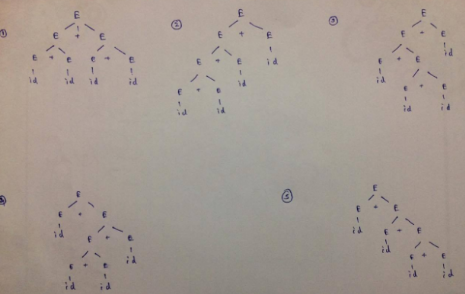Courses

# Test: Regular Expressions & Finite Automata- 3

## 20 Questions MCQ Test RRB JE for Computer Science Engineering | Test: Regular Expressions & Finite Automata- 3

Description
This mock test of Test: Regular Expressions & Finite Automata- 3 for Computer Science Engineering (CSE) helps you for every Computer Science Engineering (CSE) entrance exam. This contains 20 Multiple Choice Questions for Computer Science Engineering (CSE) Test: Regular Expressions & Finite Automata- 3 (mcq) to study with solutions a complete question bank. The solved questions answers in this Test: Regular Expressions & Finite Automata- 3 quiz give you a good mix of easy questions and tough questions. Computer Science Engineering (CSE) students definitely take this Test: Regular Expressions & Finite Automata- 3 exercise for a better result in the exam. You can find other Test: Regular Expressions & Finite Automata- 3 extra questions, long questions & short questions for Computer Science Engineering (CSE) on EduRev as well by searching above.
QUESTION: 1

### Consider the following languages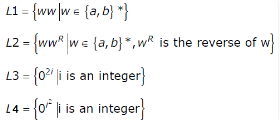Q. Which of the languages are regular?

Solution:

A language is known as regular language if there exists a finite automaton (no matter whether it is deterministic or non-deterministic) which recognizes it. So if for a given language, we can come up with an finite automaton, we can say that the language is regular. But sometimes, it is not quite obvious to design an automaton corresponding to a given language but it surely exists. In that case, we should not start thinking that the given language is not regular. We should use pumping lemma to decide whether the given language is regular or not.
According to pumping lemma, “Suppose L is a regular language, then there exists a l ≥ 1 such that for all string s ∈ L, where |s| ≥ l, we can always split s (there exists at least one such splitting) in such a way that s can be written as xyz with |xy| ≤ l and y ≠ ε  and for all i ≥ 0 , xyiz ∈ L”. l is known as pumping length. Let’s rephrase the given Lemma for non regular languages. Suppose L is a language, if for all l ≥ 1 there exist a string s ∈ L with |s| ≥ l such that for all splitting (there doesn’t exists a single splitting which doesn’t follow this rule) of s in form of xyz such that |xy| ≥ l and y ≠ ε , there exists an i≥ 0 such that xyiz ∉ L, then L is not regular. Notice that here we stress on finding such s if we want to prove that a language is not regular.
Choice of the Questions:
(a) In choice 1, Lets first consider w being of length n and containing only a. In this case the language represents anan. The length of the string represented by language should be Even. Consider l = n, then xyz = anan with xy = an. lets assume y = a, then consider the membership of xyiz with i = 0. This will simply be of odd length which doesn’t belongs to L. So L is not regular. To discuss it in more detail, let’s consider another example. Suppose w = apb, then string formed by L will be apbapb which is of length 2p + 2. Assume l = p, then xy = ap. suppose y = a, then consider the membership of xyiz with i = 0. This certainly doesn’t belongs to L. So L is not regular.
(b) In choice 2, The first example will work as above. In the second example, the string will be apbbap, and there will be no changes in process for proving it to be non regular.
(c) In choice 3, Assuming that we are considering integer from 0 and 02∗n results in empty string, Which is also accepted, We can simply construct a DFA as given below. It simply accepts a string if it is either empty or contain even number of zeros. So the language is regular.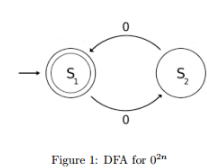(d) In choice 4, We can simply assume that the pumping length l =i2/2. Now consider the xy = 0l with y = 0, Now if we check the membership of xy2z, we can find that this will represent 0i^2+1, and corresponding to which there exists no j such that j2 = i2 + 1 where i and j are integer except j = 1 and i = 0. But since i can’t be zero. In Short, using pumping lemma, we can generate 0i^2+1 as well as 0i^2-1, which won’t be available in L. So L is not regular.

QUESTION: 2

### Let S and T be language over Σ = {a,b} represented by the regular expressions (a+b*)* and (a+b)*, respectively. Which of the following is true?

Solution:

Both have same output because if we draw DFA of S which is (a+b*)*, at final state it is just repeating.

QUESTION: 3

### Let L denotes the language generated by the grammar S -> 0S0/00. Which of the following is true?

Solution:

Option A L is not 0+ , because 0+ will contain any arbitrary string over alphabet 0 with any no of 0's ( except empty string ), for ex: {0, 00, 000,00000}, but L will only have the strings as { 00, 0000, 000000,...}, i.e only even no of  0's ( excluding empty string}.  Option D : L is a Context Free Language, because the Grammar G which generates the language L is Context Free Grammar. A Grammar G is CFG if all of its productions are of the form A->α, where A is a single non-terminal and α belongs to (V∪ T)* , i.e  α can be a string of terminals and/or Non-terminals. (V represents a non-terminal and T represents a terminal) Option C : L is a Regular Language, Because we are able to write a regular expression for it ( and also able to make a Finite Automaton), which is (00)+.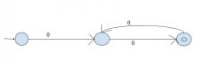Option B :  Hence This option is Correct, because L is Regular but not 0+, as we proved above

QUESTION: 4

What can be said about a regular language L over {a} whose minimal finite state automaton has two states?

Solution:

There are two states. When first state is final, it accepts even no. of a's. When second state is final, it accepts odd no. of a's.

QUESTION: 5

Consider the following Deterministic Finite Automata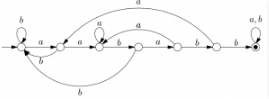Q.  Which of the following is true?

Solution:

To reach the accepting state, any string will have to go through edges having aababb as labels in order. Though it might not be a continuous substring, but it sure will be a substring. There might be some cases where same substring always exists as a prefix or suffix for some DFA, but in this situation we don’t have to consider those cases, given this question has single choice answer. − > O − a− > O − a− > O − b− > O − a− > O − b− > O − b− > O Hence, correct answer should be (B).

QUESTION: 6

How many minimum states are required in a DFA to find whether a given binary string has odd number of 0's or not, there can be any number of 1's.

Solution: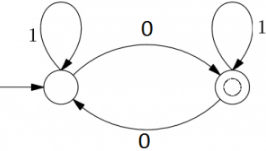QUESTION: 7

Consider the DFAs M and N given above. The number of states in a minimal DFA that accepts the language L(M) ∩ L(N) is __________.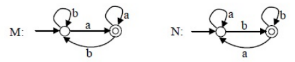Solution:

In DFA M: all strings must end with 'a'. In DFA N: all strings must end with 'b'. So the intersection is empty. For an empty language, only one state is required in DFA. The state is non-accepting and remains on itself for all characters of alphabet.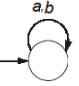QUESTION: 8

Consider alphabet ∑ = {0, 1}, the null/empty string λ and the sets of strings X0, X1 and X2 generated by the corresponding non-terminals of a regular grammar. X0, X1 and X2 are related as follows:

X0 = 1 X1
X1 = 0 X1 + 1 X2
X2 = 0 X1 + {λ}

Q. Which one of the following choices precisely represents the strings in X0?

Solution:

The smallest possible string by given grammar is "11". X0 = 1X1
= 11X2 [Replacing X1 with 1X2]
= 11 [Replacing X2 with λ]
The string "11" is only possible with option (C).

QUESTION: 9

Which of the following languages is/are regular?

L1: {wxwR ⎪ w, x ∈ {a, b}* and ⎪w⎪, ⎪x⎪ >0} wR is the reverse of string w
L2: {anbm ⎪m ≠ n and m, n≥0
L3: {apbqcr ⎪ p, q, r ≥ 0}

Solution:

L3 is simple to guess, it is regular. Below is DFA for L1.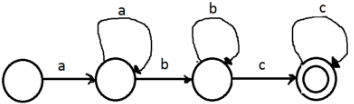L1 is interesting. The important thing to note about L1 is length of x is greater than 0, i.e., |x| > 0.
So any string than starts and ends with same character is acceptable by language and remaining string becomes w. Below is DFA for L1.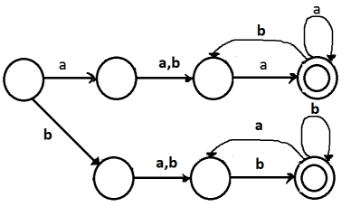QUESTION: 10

The number of states in the minimal deterministic finite automaton corresponding to the regular expression (0 + 1)*(10) is ____________

Solution:

Below is NFA for the given regular expression (0 + 1)*(10)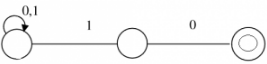Below is DFA for the same.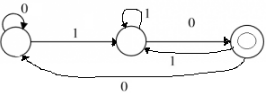QUESTION: 11

Let T be the language represented by the regular expression Σ∗0011Σ∗ where Σ = {0, 1}. What is the minimum number of states in a DFA that recognizes L' (complement of L)?

Solution:

The given regular expression matches with all strings that contain 0011. The complement should match with all strings except the strings with 0011 as substring. Following are some interesting facts/observations from this question.
1) Complement of a regular language is also regular.
2) Since complement is regular, it is always possible to make a DFA for complement.
3) A DFA that accepts its complement is obtained from the above DFA by changing all non-accepting states to accepting states and vice versa as done in this question. Below is DFA for the complement. We can get below DFA by first drawing DFA of regular language for strings with substring as 0011. After drawing DFA, we can invert all states (single circle to double circle and vice versa)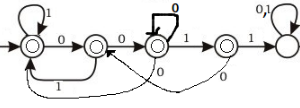QUESTION: 12

Which one of the following regular expressions is NOT equivalent to the regular expression (a + b + c) *?

Solution:

C- (ab)* + c*)* will always give strings with "ab" together.Whereas (a+b+c)* would generate language where a,b,c may not be always together. A,B,D may generate same language as (a+b+c)*   So, Answer is (C)

QUESTION: 13

Which one of the following strings is not a member of L (M)?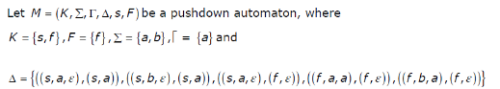Solution:

Basics of PDA A push down automata or a PDA is essentially a NFA with a stack and its transition function also depends on the symbol at the stack top. Formally, a PDA is a 6-tuple (Q, Σ, Γ, δ, q0, F) with Q being set of all possible states, Σ is the set of all possible input, Γ is the set of all possible stack symbol, δ : Q × Σ × Γ → Q × Γ is the transition function, q0 is the initial state, and F ⊆ Q is the set of final state. Some times, PDA is also known to be of 7 tuples when we considers the initial stack symbol z0 as an additional member in the above 6 tuples. Note that there should exists a transition function for a given input otherwise there won’t be any possible move. The PDA can be of two types: Empty stack accepting PDA and Final state PDA. Empty stack accepting PDAs are the PDAs for which a given input is accepted if on a given input string, when the input exhausts, the stack should be empty. Similarly, a Final state accepting PDA is a PDA for which a given input is accepted if on a given input string, when the input exhausts, thePDA should be in one of the final states. A Non-deterministic PDA or NPDA is a PDA in which, for a given input, multiple output is possible. i.e. The Transition function δ maps a given input from Q × Σ × Γ to a finite set of Q × Γ. For the given question, since there is a more than one output corresponding to a given input for transition function, we will consider a NPDA for the execution of the input and we will halt our execution for a corresponding branch if there is no move possible for a given input and We assume that if the machine halts at one of the final state and input is exhausted, we accept the string otherwise we reject it. Notice, the Given PDA is final state accepting PDA and not the empty stack accepting PDA. Choice of the Questions:  We will assume the initial stack symbol is ε, and
• In choice 1, The execution will be as follow: (s, a, ) → (s, a) or (f, ε). Let’s first consider, (s, a) as output and execute the remaining input. (s,a, a) → No valid Move. halt this branch and not in the final state, so not accepted. Now consider (f, ε) as output. (f, a, ε) → No Valid Move. halt this branch Notice that the PDA is in one of the final state, But the input string is not exhausted, So this should not be accepted by the PDA.
• In choice 2, The Execution will start as of choice 1 and will halt at character 2 as no possible move. This option is also not accepted in same way as of the first option. 3
• In choice 3, The execution will be as follow: (s, b, ε) → (s, a). (s, a, a) → No Possible move. halt this branch. Since halted before reaching to any final state so this option is not accepted.
• In choice 4, This option is also not accepted as the PDA will halt at second character as it halted in previous option. This option is also not accepted.   Assuming that if the PDA halts on a input due to no possible move and if the current state is one of the final state but the input is not exhausted, PDA will reject that string, For Question 2, Every answer is true i.e. None of the given for string belongs to the given PDA’s language. Again, Assuming that if the PDA halts on a input due to no possible move and if the current state is one of the final state but the input is not exhausted, the PDA will keep on consuming the input string until the end of string comes, string in option (A) and option (B) will be accepted by the language of this PDA. while option (C) and option (D) still won’t get accepted as by the end of the string, the PDA won’t be in final state. So keeping this assumption in mind, option (C) and option (D) both will be true for this question.

QUESTION: 14

Let L be a regular language and M be a context-free language, both over the alphabet Σ. Let Lc and Mc denote the complements of L and M respectively. Which of the following statements about the language Lc∪ Mc is TRUE

Solution:

Proposition: L is a regular language M is a context free language Derivation: L_c  union M_c = complement{L intersection M} Now, L intersection M is a CFL according to closure laws of CFLs, i.e. intersection of a CFL with RL is always a CFL. But, complement{L intersection M} might not be a CFL because complement over CFL doesn't guarantee a CFL. It can even be a RL or it might even lie outside the CFL circle. It will be a context-sensitive language certainly, but nothing else can be said about it. Conclusion: Considering the above derivation, none of the statements are true. Hence correct answer would be (D) None of the above.

QUESTION: 15

Which of the following statements is TRUE about the regular expression 01*0?

Solution:

First of all, A string can never be infinite because String is a finite sequence of symbols over Σ So option (c) and (d) are eliminated. And because of star(*) it can generate infinite set. So Option (B) is CORRECT.

QUESTION: 16

The language {0n 1n 2n | 1 ≤ n ≤ 106} is

Solution:

The value of ‘n’ is finite. So, only finite number of strings can be part of given language. Therefore, we can construct a finite state automata for this language.

Thus, option (A) is correct.

Please comment below if you find anything wrong in the above post.

QUESTION: 17

Consider the non-deterministic finite automaton (NFA) shown in the figure.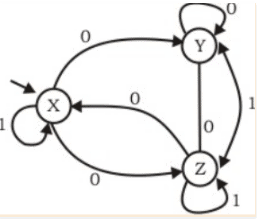State X is the starting state of the automaton. Let the language accepted by the NFA with Y as the only accepting state be L1. Similarly, let the language accepted by the NFA with Z as the only accepting state be L2. Which of the following statements about L1 and L2 is TRUE? Correction in Question: There is an edge from Z->Y labeled 0 and another edge from Y->Z labeled 1 - in place of double arrowed and no arrowed edges.

Solution:

A generic approach to solve such questions would be to come up with the corresponding regular languages and compare the two. Ardens theorem could be used to achieve the objective. Moreover, some conclusions could be drawn without even deriving the languages completely, solely from the intermediary equations. Constructing equations for every state -: X = Z0 +X1, Y = X0 +Y0 +Z0 && Z = X0 + Y1 + Z1. Clearly, regular languages L1 and L2 couldn’t be same. Now, to check which of the other three options are correct, we need to find : 1) a string which is accepted by L1 but not by L2. 2) a string which is accepted by L2 but not by L1. If there exists string 1) but not 2) then L2 ⊂ L1 and likewise. Trying our hands at the given DFA, 1) string 00 is accepted by L1 but not by L2 and 2) string 01 is accepted by L2 but not by L1. Hence, neither of the two languages our subset of the other.

L1 can have 00 string while L2 can't. L2 can have 01 while L1 can't None of them can be either equal or proper subset of each other.So Ans. D.

QUESTION: 18

A language L satisfies the Pumping Lemma for regular languages, and also the Pumping Lemma for context-free languages. Which of the following statements about L is TRUE?

Solution:

Pumping lemma is negativity test. We use it to disprove that a languages is not regular. But reverse is not true.

QUESTION: 19

Consider the context-free grammar E → E + E E → (E * E) E → id
where E is the starting symbol, the set of terminals is {id, (,+,),*}, and the set of nonterminals is {E}.

Q. Which of the following terminal strings has more than one parse tree when parsed according to the above grammar?

Solution:

According to leftmost derivation :
E -> E + E (using E -> E + E) E -> E + E + E (using E -> E + E) E -> E + E + E + E (using E -> E + id) E -> id + E + E + E (using E -> id) E -> id + id + E + E (using E -> id) E -> id + id + id + E (using E -> id) E -> id + id + id + id

According to rightmost derivation :
E -> E + E (using E -> E + E) E -> E + E + E (using E -> E + E) E -> E + E + E + E (using E -> E + id) E -> E + E + E + id (using E -> id) E -> E + E + id + id (using E -> id) E -> E + id + id + E (using E -> id) E -> id + id + id + id

Thus, option (A) is correct.

Please comment below if you find anything wrong in the above post.

QUESTION: 20

Consider the context-free grammar E → E + E E → (E * E) E → id
where E is the starting symbol, the set of terminals is {id, (,+,),*}, and the set of non-terminals is {E}. For the terminal string id + id + id + id, how many parse trees are possible?

Solution:

Background Required to solve the question - Parse Tree Construction.

Explanation : In order to produce the yield id + id + id + id , we only required 3 productions of type E → E + E as 3 ‘+’ are required in the final string. This can be done in 5 ways as shown in the picture given below: